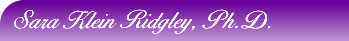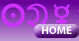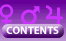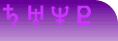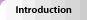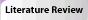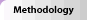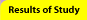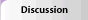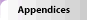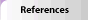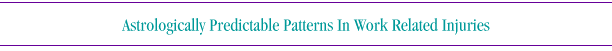### Results of the Study

This chapter presents and explains the data which were obtained for the purpose of this study. It includes the results obtained following the statistical computation of the data and addresses all the findings relevant to the research hypothesis and research questions. This is further supported by tables and figures to provide a visual summary of sets of related data from this research.

#### Data Collection:

The target population for this study consisted of individuals who were injured in a work related injury and filed a Workers' Compensation claim through an attorney, who then referred them for a medical-legal evaluation. All subjects were injured severely enough to be disabled for a minimum of three months.

Two samples were obtained for this study: Sample A consisted of 523 injury cases which were provided by three independent medical-legal transcribers who processed evaluations for a Los Angeles medical clinic. This sample included birth dates and dates of injury for each subject. No demographics other than age for each subject were available and there was no separation to male and female groups. No interpreter was used for the evaluation, indicating that all subjects were English speaking. The injuries for this sample occurred between the years 1983 and 1991. Sample A finally consists of only 414 subjects as it was discovered that some of the subjects (109) were inadvertently reported twice. This sample excluded all cuspal birth dates and cuspal dates of injury before processing. (Cuspal dates are dates when the Sun is changing Sun sign, and because no exact birth times were available, cuspal dates were eliminated in all samples).

Sample B consisted of 609 subjects obtained directly from a Los Angeles clinic that evaluates and treats Hispanic (mainly Mexican and some El Salvadorean) injured workers. (All evaluations were done with the help of a Spanish speaking interpreter). A clinic staff member copied the required information from the clinic's files according to the same instructions that the medical-legal transcribers were asked to follow, with one addition: A separation into male and female subjects was recorded. Injury years for this sample ranged from 1981 to 1992. At no time did the researcher meet or see any of the subjects for either sample. Sample B was divided into Sample B/Females (N=126), and Sample B/Males (N=483). Six cuspal birth dates were eliminated from the female population of Sample B for the analysis by Sun sign, thus using only 120 females for that particular analysis. All other computations were done on the entire sample. From Sample B/males, 13 cuspal birth dates were eliminated for the same analysis, using only 470 subjects for the Sun sign distribution.

A replication sample of 55 subjects (Sample C) was obtained during the process of reviewing the literature, as C.E.O. Carter (1932) provides birth dates and dates of injury for 55 of his reported 168 cases. These were all birth dates with exact birth times, so no cuspal deletions were needed. All injury dates were prior to the year 1929.

A control group of completed suicide subjects (Sample D) was provided by David Lester (1987). These were recorded suicide subjects who committed suicide in Philadelphia during the year of 1982. A total of 206 subjects had full birth dates and dates of suicide and were included in the sample. However, 45 of these were cuspal birth dates (21) or cuspal suicide dates (24),and were eliminated from the Sun sign computations. They were, however, included in all other computations. No separation into male/female sub-groups was conducted for this sample, as it was used as a control for the entire research sample of 1023 subjects (Sample A + B).

In order to ensure that the research samples A and B are a good representation of the entire population of injured persons in California, a comparison by age groups was conducted. Percentages of people in each age group were compared with the statistics provided by the state of California (Appendix B). The results are tabulated in Tables 1 - 4 and Figures 1 - 4 below. The tables list the age groups of the subjects in each sample, allot an age category to each age group, list the actual number of subjects in each category and the percentage of the particular sample that were in each age group. Figures 1 - 4 plot the distribution of the age groups by percentage in each sample against the distribution of age groups by percentage for the state of California (Appendix B). Table 1 and Figure 1 describe Sample A with 414 subjects. Table 2 and Figure 2 describe Sample B/females with 126 subjects. Table 3 and Figure 3 describe Sample B/males with 483 subjects, and Table 4 and Figure 4 describe the combined samples A and B with 1023 subjects.

It is obvious from these tables and figures, that the samples are a good approximation by age distribution to the distribution by age of the injured population in California for the year 1989, especially when the entire sample (N=1023) is plotted (Table 4 and Figure 4).

 Table 1 Figure 1 Table 2 Figure 2 Table 3 Figure 3 Table 4 Figure 4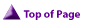#### Research Hypothesis:

According to the research hypothesis it was expected that significantly more people are injured when the transiting Sun forms a hard aspect (conjunction or 0°, square or 90°, and opposition or 180°) to the natal Sun than would be expected by chance. This hypothesis was analyzed from two perspectives: a) Sun sign separation, and b) separation by degree.

Sun sign separation: Tables 5-11 and Figures 5-11 present the analysis of the data for all research, replication and control samples according to Sun sign separation. The tables list the injury categories, the number of subjects in each injury category and the expected value for each injury category. The expected value was derived by dividing the sample size (N) by the number of categories (12). The expected value indicates how many people are expected to be in each category if the injuries occurred at random. The expected value is used in the computation of the Chi-Square for Goodness of Fit, which is the statistical test used in this study. Below each table are the obtained Chi-Square values, degrees of freedom used and the level of statistical significance for each sample. Figures 5 - 11 are the graphic representations of the data in each Table.

 Table 5 Figure 5 Table 6 Figure 6 Table 7 Figure 7 Table 8 Figure 8 Table 9 Figure 9 Table 10 Figure 10 Table 11 Figure 11

Table 5 and Figure 5 present the results for Sample A (N=414). Sample A did not include cuspal births. Note that the expected value in each injury category is 34.5. Only injury category 5 (34), 6 (34) and 8 (35) fall around the expected value. All hard aspect categories (1, 4, 7, and 10) are significantly higher than the expected value, and all the other categories are much lower than the expected value, especially categories 3 (19) and 11 (24). The Chi-Square value with 11 degrees of freedom for this test was 31.56 and the statistical significance of this distribution is P< .001 (P=0.00089).

Table 6 and Figure 6 present the results for Sample B/females (N=120). Six subjects were deleted from the entire female group of 126 because of cuspal birth dates or injury dates. The expected value for each injury category for this sample is 10. Note here, too, that only injury categories 5 (11) and 8 (9) are around the expected value, while the hard aspect categories (1, 4,7, and 10) are all considerably higher in value. The lowest observed values are category 3 (6) and category 12 (5). The Chi-Square value with 11 degrees of freedom for this test was 19.8, and the statistical significance of this distribution is P< .05 (P=0.048).

Table 7 and Figure 7 present the results for Sample B/males (N=470). A total of 13 cuspal birth dates and injury dates were deleted from this sample. The expected value for each injury category in this sample is 39.16. Only categories 2 (38) and 11 (36) are close to the expected value, whereas the hard aspect categories (1, 4, 7, and 10) are significantly higher. Categories 8 (22) and 12 (20) are strikingly low in this sample. The Chi-Square value with 11 degrees of freedom for this test was 76.1917, and the statistical significance of this distribution is P< .00000001 (The actual P value has more than seven zeros following the decimal point).

Table 8 and Figure 8 represent the results for the entire Sample B (N=590). A total of 19 cuspal births were eliminated from the sample as seen above in Sample B/females and Sample B/males. The expected value for each injury category in this sample is 49.16. The two injury categories that are closest to the expected value are 2 (45), and 11 (43). The hard aspect categories (1,4, 7, 10) are well above the expected value, while the two lowest injury categories are 8 (31) and 12 (25). The Chi-Square test value for this sample with 11 degrees of freedom is 89.29 and the statistical significance of this distribution is P< .000000001 (The actual P value has more than nine zeros following the decimal point).

Table 9 and Figure 9 represent the results for the Samples A and B combined (N=1005). A total of 19 cuspal births were eliminated from the sample as seen above in Sample B/females and Sample B/males. The expected value for each injury category in this sample is 83.75. The two injury categories that are closest to the expected value are 2 (72), 5 (72), and 6 (72). The hard aspect categories (1, 4, 7, 10) are well above the expected value, while the two lowest injury categories are 3 (55) and 12 (55). The Chi-Square test value for this sample with 11 degrees of freedom is 107.728 and the statistical significance of this distribution is P< .0000000001.

Table 10 and Figure 10 depict the results for replication Sample C, derived from C.E.O. Carter's book (1932). These are 55 injured people who were listed in the above book as having accurate birth dates and dates of injury. As all of Carter's sample had accurate times of birth, there was no need to eliminate any cuspal birth dates. The expected value for each category in this sample (N/12) is 4.58. Injury categories 12 (4) and 9 (5) are close to the expected value. Categories 1 (12), 4 (12) and 7 (7) are well above the expected value (and are the only ones that are above it), while category 10 (2) is well below the expected value, although it is one of the hard aspects. The lowest observed values in the injury categories are 2 (1) and 5 (1). The Chi-Square test value with 11 degrees of freedom is 36.008, with a statistical significance of P< .0002 (P=0.00016).

Table 11 and Figure 11 describe the results for Sample D, the control group obtained from Lester's (1987) suicide study (N=161). A total of 206 suicide cases were obtained but 45 cuspal birth dates and dates of injury had to be deleted from this sample. The expected value for each injury category in this sample is 13.41. Categories 1 (14), 5 (14), 7 (13), 9 (13) and 12 (14) are all close to the expected value. Categories 4 (15), 6 (15), and 11 (16), are slightly above the expected value, while category 2 (11) is the lowest below the expected value. The Chi-Square test with 11 degrees of freedom yielded a value of 1.858 and a P=0.9989, indicating that this sample is practically randomly distributed according to the expected values.Separation by degree: Tables 12-18 present the analysis of the data for all research, replication and control samples according to separation by degree. Three orbs were used: An orb of 15° on either side of the exact hard aspect (345°-15° for the conjunction; 75°-105° for the first square, 165°-195° for the opposition, and 255°-285° for the second square) was tabulated for each sample and is listed in the first column of each one of Tables 12-18. A 10° orb on either side of the exact hard aspect (350°-10° for the conjunction, 80°-100° for the first square, 170°-190° for the opposition, and 260°-280° for the second square) was tabulated for each sample and is listed in the second column of each one of Tables 12-18. A 5° orb on either side of the exact hard aspect (355°-5° for the conjunction, 85°-95° for the first square, 175°-185° for the opposition, and 265°-275° for the second square) was tabulated for each sample and is listed in the third column of each one of Tables 12-18. Each column respectively lists the following: The number of subjects observed in each orb category, the expected value for that category, the Chi-Square value, the degrees of freedom used, and the statistical significance value (P value). The expected number was derived as follows: The 15° orb covers 30° for each hard aspect, thus accounting in total for 120 degrees, which is 33.33 of the entire circle. The 10° orb covers 20° for each hard aspect category, totaling 80° of the entire circle, which is 22.22 of the circle, and the 5° orb covers 10° for each hard aspect category, resulting in 11.11 of the circle. So, the expected value for each category would be 33.33, 22.22 and 11.11 respectively, of the number of subjects observed, i.e. N x 33.33, N x 22.22, and N x 11.11. The Chi-Square test for Goodness of Fit was done with 1 degree of freedom (a 2 x 2 tabulation). Note that the sample sizes for Tables 12-16 include all subjects without any deletions because only the degree of separation was computed while no consideration was given to the zodiacal (Sun sign) position of the Sun either at the time of birth or at the time of the injury. Tables 17 and 18 represent the same tabulation for Sample C (the Carter replication group) and for Sample D (the suicide control group).Table 12 represents Sample A and records 178 observed hard aspects to the natal Sun from the transiting Sun by 15° orb. The expected value is 138 (33.33 of 414). The Chi-Square value with one degree of freedom is 17.3913, and the statistical significance of this value is P=0.00003. 120 hard aspects to the natal Sun from the transiting Sun by 10° orb are listed in the center column. The expected value is 92 (22.22 of 414). The Chi-Square value with one degree of freedom is 10.9565, which yields a statistical significance of P=0.000932. The last column lists 66 observed hard aspects to the natal Sun from the transiting Sun by 5° orb. The expected value is 46 (11.11 of 414). The Chi-Square value with one degree of freedom is 9.782, which yields a statistical significance of P=0.00176. Table 13 represents Sample B/females, and records 61 observed hard aspects to the natal Sun from the transiting Sun by 15° orb. The expected value is 42 (33.33 of 126). The Chi-Square value with one degree of freedom is 12.8929 and the statistical significance of this value is P=0.00033. 39 hard aspects to the natal Sun from the transiting Sun by 10° orb are listed in the center column. The expected value is 28 (22.22 of 126). The Chi-Square value with one degree of freedom is 5.556, with a P value of 0.0184. The last column lists 19 observed hard aspects to the natal Sun from the transiting Sun by 5° orb. The expected value is 14 (11.11 of 126). The Chi-Square value with one degree of freedom is 2.008, which yields a P value of 0.156, which is only marginally significant statistically. Table 14 represents Sample B/males and records 232 observed hard aspects to the natal Sun from the transiting Sun by 15° orb. The expected value is 161 (33.33 of 483). The Chi-Square value with one degree of freedom is 46.9658, and the statistical significance is P< .0000001. 171 hard aspects to the natal Sun from the transiting Sun by 10° orb are listed in the center column. The expected value is 107 (22.22 of 483). The Chi-Square value with one degree of freedom is which yields a statistical significance of P< .0000000001. The last column lists 104 observed hard aspects to the natal Sun from the transiting Sun by 5° orb. The expected value is 54 (11.11 of 483). The Chi-Square value with one degree of freedom is 52.124, which yields a statistical significance of P< .0000001. Table 15 represents Sample B (males and females combined) and records 293 observed hard aspects to the natal Sun from the transiting Sun by 15° orb. The expected value is 202 (33.33 of 609). The Chi-Square value with one degree of freedom is 61.392, and the statistical significance P< .00000001. 210 hard aspects to the natal Sun from the transiting Sun by 10° orb are listed in the center column. The expected value is 135, (22.22 of 609). The Chi-Square value with one degree of freedom is 53.559, which is statistically significance at P< .0000001. The last column lists 123 observed hard aspects to the natal Sun from the transiting Sun by 5° orb. The expected value is 67 (11.11 of 609). The Chi-Square value with one degree of freedom is 52.603, which yields a statistical significance of P< .0000001. Table 16 represents Sample A and B combined, and records 471 observed hard aspects to the natal Sun from the transiting Sun by 15° orb. The expected value is 341 (33.33 of 1023). The Chi-Square value with one degree of freedom is 74.34, with a statistical significance of P< .0000001. 330 hard aspects to the natal Sun from the transiting Sun by 10° orb are listed in the center column. The expected value is 227 (22.22 of 1023). The Chi-Square value with one degree of freedom is 60.064, which is statistically significant at P<.0000001. The last column lists 189 observed hard aspects to the natal Sun from the transiting Sun by 5° orb. The expected value is 114 (11.11 of 1023). The Chi-Square value with one degree of freedom is 55.53, which yields a statistical significance of P< .0000001. Table 17 represents Sample C (Carter), and records 28 observed hard aspects to the natal Sun from the transiting Sun by 15° orb. The expected value is 18 (33.33 of 55). The Chi-Square value with one degree of freedom is 8.2583, and the statistical significance of this value is P=0.00405. 23 hard aspects to the natal Sun from the transiting Sun by 10° orb are listed in the center column. The expected value is 12, (22.22 of 1023). The Chi-Square value with one degree of freedom is 12.897, which yields a statistical significance of P=0.00033. The last column lists 17 observed hard aspects to the natal Sun from the transiting Sun by 5° orb. The expected value is 6 (11.11 of 55). The Chi-Square value with one degree of freedom is 22.636, which yields a statistical significance of P=0.0000019. Table 18 represents Sample D (Suicide control group), with 67 observed hard aspects to the natal Sun from the transiting Sun by 15° orb. The expected value is 68 (33.33 of 206). The Chi-Square value with one degree of freedom is 0.021952, and P=0.8822. 49 hard aspects to the natal Sun from the transiting Sun by 10° orb are listed in the center column. The expected value is 46 (22.22 of 206). The Chi-Square value with one degree of freedom is 0.2519, which yields a P=0.6157. The last column lists 28 observed hard aspects to the natal Sun from the transiting Sun by 5° orb. The expected value is 23 (11.11 of 206). The Chi-Square value with one degree of freedom is 1.2236, which yields a P=0.2686. Note that none of the P values in Sample Dare significant. Table 19 compares the results for all research samples when all the hard aspect injury categories were combined and compared as a combined score against the rest of the injury categories. Categories 1, 4, 7, and 10 (the hard aspect categories) comprise 33.33 of the 12 categories, thus, an expected value is 33.33 of the N value. The first column lists the observed value of all the hard aspects in Sample A (N=414) and places it at 186. Below that is listed the expected value of 138 (414 x 33.33). The Chi-Square value with 1 degree of freedom is 25.04, and the statistical significance is P=0.00000012. The second column lists the observed value for all the hard aspects in Sample B/females (N=120) at 61, with an expected value of 40. The Chi-Square value with 1 degree of freedom is 16.53, and the statistical significance is P=0.000047. The third column lists the observed value for all hard aspects in Sample B/males (N=470) at 236, with an expected value of 157. The Chi-Square value with 1 degree of freedom is 59.7, with a statistical significance of P< .00000001. The fourth column lists all the observed value the hard aspects in Sample B (Combined male and female, N=590) at 297, with an expected value of 197. The Chi-Square value with 1 degree of freedom is 76.2, statistically significant at P< .00000001. The last column lists the observed value for all the hard aspects for the entire research population, Sample A and Sample B combined (N=1005) at 483, with an expected value of 335. The Chi-Square value with 1 degree of freedom is 86.2, statistically significant at P< .0000000001. This table indicates that when all hard aspects are taken together, the significance of the distribution is even higher than when the injury categories were treated separately (Tables 5 - 11).During the process of analyzing the data, several people were asked by the researcher to form an opinion as to the possible reasons for the distributions found. They were not told that an astrological pattern was being investigated. They all answered without hesitation, that being injured around one's birthday could be expected for reasons such as being drunk, being tired from too many parties, general excitation around the birthday, and lowered spirits due to the feeling of getting older, etc. No one could, however, suggest an alternative explanation for the other three hard aspects, i.e., the two squares and the opposition. In order to measure the strength of the hard aspect effect without the birthday (conjunction), further computation was done on all samples, for the remaining 11 injury categories (Table 20) and the three hard aspect categories combined (i.e., categories 4, 7, and 10 only) without the conjunction (Table 21). Table 20 sums up the results of this computation for all research samples. The first column lists the results for Sample A. Note that N=365, as injury category 1 was removed from the sample (See Table 5). The new expected value in each cell is now 365/11=33.18, and again, the Chi-Square test for Goodness of Fit was performed on the remaining 11 injury categories with 10 degrees of freedom, and yielded a value of 26.21. The statistical significance of this computation is P=0.0034. The second column lists the results for Sample B/females with N=105. (See Table 6). The new expected value in each cell is now 105/11=9.5, and the Chi-Square value with 10 degrees of freedom is 19.974. The statistical significance is P=0.055. The third column lists the results for Sample B/males with N=395 (See Table 7). The new expected value in each cell is now 395/11=35.9. The Chi-Square value with 10 degrees of freedom is 44.092, with a statistical significance of P=0.0000031. The fourth column lists the results for Sample B (both male and female) with N=500 (See Table 8). The new expected value in each cell is now 500/11=45.5. The Chi-Square value with 10 degrees of freedom is 56.5, at a statistical significance of P< .0000001. The last column lists the results for the entire research population, (Samples A and B combined) with N=866 (See Table 9). The new expected value in each cell is now 866/11=72.237. The Chi-Square value with 10 degrees of freedom is 78.7, statistically significant at P< .000000001. So, it is obvious from this computation, that the research hypothesis is strongly supported even without the conjunction category. Table 21 repeats the computation of all research samples looking at the combined values of all hard aspect categories (without the conjunction) compared with the remaining categories together much like the tabulation depicted in Table 19. The difference here is in the combined group of categories 4, 7 and 10 compared with the rest of the categories excluding category 1 (the conjunction or birthday category). The combined three categories (4, 7, and 10) comprise 27.27 of the entire sample, therefore the expected value for the Chi-Square test is N x 22.27. The first column represents the results for Sample A (N=365). The observed value for categories 4, 7 and 10 is 137. The expected value is 99.5. The Chi-Square value with 1 degree of freedom is 20.014 and the statistical significance is at P=0.0000079. The second column represents the results for Sample B/females (N=105). The observed value for categories 4, 7 and 10 is 46 and the expected value is 28.6. The Chi-Square value with 1 degree of freedom is 13.77 with a statistical significance of P< =0.0002. The third column describes the results for Sample B/males (N=395). The observed value is 161 and the expected value is 108. The Chi-Square value with 1 degree of freedom is 35.8, statistically significant at P< .000001. The fourth column represents the results of the entire Sample B (N=500). The observed value is 207 with an expected value of 137. The Chi-Square value with 1 degree of freedom is 49.26, statistically significant at P< .0000001. The last column represents the results for the entire research population, Samples A and B combined (N=866). The observed value is 344 and the expected value is 236. The Chi-Square value with 1 degree of freedom is 67.93, statistically significant at P< .00000001. This table also provides clear evidence in the direction of the research hypothesis even when category 1 (the conjunction) that represents the injury date around one's birthday is removed from the computations, and this is true for all samples and all categories.#### Research Questions:

One research question in this study deals with the differences between male and female populations in regards to the research hypothesis, based on the theoretical premise that the Sun is a significator of men or males in general (Hand, 1976:47). While it was impossible to obtain a separation into male/female subjects in Sample A, it was done in Sample B and resulted in 126 female injuries and 483 male injuries. Table 2 and Figure 2 represent the distribution by age of the 126 women, Table 6 and Figure 6 represent the distribution by injury category and the statistical significance of the distribution (P< .05), and Table 13 addresses the same sample from the point of view of degree separation and orbs. Tables 19, 20 and 21 depict additional computations across all samples and the second column in all of them give the results for Sample B/females. All results on the female group (Sample B/females) except the 5° orb category were statistically significant in the direction of the research hypothesis, indicating that there is no difference between males and females regarding proneness to injury when the transiting Sun aspects the natal Sun by a hard aspect.

Another research question tried to find whether there is another aspect from the transiting Sun to the natal Sun that is prominent in these injuries. As seen in Tables 5 - 11, only the hard aspect categories were larger than expected by chance, which is the direction of the research hypothesis, and therefore, no other aspect from the transiting Sun was found to be prominent in any of the research samples.

This study hoped to find some clarifications about the strength or frequency of applying and separating aspects. An applying aspect is one that has not yet formed (i.e., when the degree separation is smaller than 90° for the square, smaller than 180° for the opposition, etc.), and a separating aspect is one where the aspect has already occurred but it is still within orb of operation (more than 90° for the square, more than 180° for the opposition, etc.). As no exact birth times were available for this study, the results for this tabulation can only be seen as good approximations.

Table 22 lists the distribution of all hard aspects (a total of 483) in three categories: Applying aspects, exact aspects, and separating aspects. The totals are given in the next to last row. It can be seen that the values for the overall number of applying aspects (222) is very close to the number of overall separating aspects (216), and therefore, from this study it is obvious that no difference was found between the two kinds of aspects. However, the exact aspect category is most interesting. The observed value is 45, when the expected value for this sample size is 11. (Four exact hard aspects are expected for each 365 people, therefore for 1023 people the expected value is 11). The observed value is more than four times larger than the expected value. The Chi-Square value with 1 degree of freedom is 106.23 and the significance level for this finding is P< .0000000001.Although there are no uniform norms for the distribution of Sun signs in the general population, and these vary according to geographical location, socio-economic factors, and eras (Dean, 1977), a computation was done on the research samples for Sun sign at birth and Sun sign at the time of injury. The CCRS Horoscope Program (Pottenger, 1992) computed birth signs for all subjects by assigning a Noon (G.M.T.) birth, therefore no deletions for cuspal births were done for this calculation, which, due to this fact, is only a close approximation (remembering that 19 cuspal births were deleted in the previous calculations).

Table 23 lists the number of subjects born in each Sun sign for each sample. Table 24 lists the number of subjects injured in each Sun sign for each sample. No tests were done on these distributions, as there are no norms to compare them with. Figure 12 plots the distribution of Table 23, and Figure 14 plots the distribution of Table 24. Figure 14 compares the overall distribution for the entire sample: One line plots for N=1023, distribution of Sun signs at birth, and the other line plots the same sample for Sun signs of the injury.

Table 23 and Figure 14 indicate that there were more people born under the Sun sign of Leo (July 23 -August 23) in this sample than any other sign (103), and that Scorpio births (October 22 - November 22) were the least frequent (73).

Table 24 and Figure 14 indicate that Aquarius (January 19 - February 19) was the Sun sign when the highest number of people were injured in this sample (100), and Sagittarius (November 22 - December 21) was the Sun sign when the lowest number of people (66) were injured. No other conclusions were drawn from this distribution, and as stated above, these results are only descriptive in nature.

In the process of analyzing the data through Pottenger's (1990) Frequencies of Aspect Research computer program, some additional findings were noted, but as they are not within the scope of this study, they will require further analysis at a later time. It was found, for example, that the next highest number of hard aspects from the transiting Sun to any natal planet was to the planets Jupiter and Saturn and to the astroid Vesta when the 5° orb was looked at.

In summary, this chapter presented the research hypothesis and the research questions, the steps in data analysis and the outcomes of the analyses. In reference to the research hypothesis, it offered evidence that in the samples studied, people tended to be injured on the job on or around their birthday, three months before or after the birthday, and six months after the birthday. In reference to the research questions, no significant difference in injury patterns was found between the male and female groups, and no significant difference was found between the applying and separating aspects. The exact aspects, were, however very high compared to the expected values. All orb categories, starting from 15° orb, through 10° and 5°, were found to be significant. The research analyses fully supported the research hypothesis. The results are discussed in detail in Chapter Five.Introduction | Literature Review | Methodology | Results | Discussion | Appendices | References